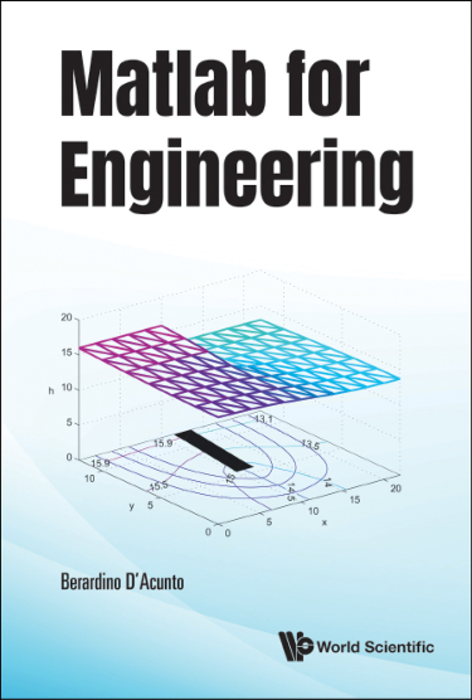News Release

# A one-stop guide to using Matlab to problem-solve

New book by Professor of Mathematical Physics gives step-by-step guidance on how to apply and assemble Matlab commands to solve problems

Book Announcement

World Scientificimage: Cover for "Matlab for Engineering" view more  Credit: World Scientific

Why write a book on Matlab that requires no prior knowledge? The statement “without prior knowledge” can be interpreted in different ways. For example, the Greek philosopher Plato preferred teaching students without pre-existing knowledge. Indeed, he claimed that the students with previous knowledge needed to do a double job when learning, beginning with the uprooting of the “wrong” knowledge that had been implanted in their minds. Of course, in the case of this book the meaning is easier. Many readers appreciate self-contained books which require no external knowledge or information.

Professor Berardino D'Acunto’s teaching experience at the University of Naples caused him to realized that engineering students did not appreciate a mere exposition of Matlab commands. He noted that they preferred to learn promptly, how to assemble the commands into programs that could solve the mathematical problems they were interested in. Matlab for Engineering written with this in mind.

In this book, after a general introduction to the essential Matlab commands mainly devoted to the matrix manipulation. The other commands are presented in action, within programs aimed at solving specific problems. Since physical processes occur very often in space and time, and the related governing equations are partial differential equations, most Matlab programs in the book are devoted to solving this kind of equations. Both the Finite Element Method and Finite Difference Method are introduced and applied. Generally, a problem is discussed globally, where the mathematical model describing the physical phenomena is derived and the equations are solved with Matlab, in a step-by-step approach.

While the this book is primarily targeted at teaching Matlab to engineering students and engineers, the book can also be useful for students, technicians and researchers working in any scientific area that could use the application of Matlab to solve problems arising from their areas of study, work and research.

Matlab for Engineering retails for US\$78 / £70 (hardcover) and is also available in electronic formats. To order or know more about the book, visit http://www.worldscientific.com/worldscibooks/10.1142/12380.

###